# The Effect of Confining Potentials on the Structure and Localization

0
1091## Abstract

Anderson localization is the phenomenon in which particles in a quantum state are unable to spread out into the rest of the system. The effects of exponential decay in normal Anderson localization are well-established; however, the effects of varying the confining potential and changing the strength of the system’s disorder to the degree of decay remain relatively untouched. We probe this through a Python simulation of a one-dimensional, non-interacting quantum system. Here we report that varying the confining potential and the degree of the system’s randomness both lead to a decrease in the degree of the decay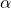< 2.

## Introduction

Quantum mechanics is a fundamental theory in physics that describes the behavior of light and matter at the atomic and subatomic levels. This fundamental theory slowly emerged from other theories to explain observations that classical physics could not explain. Ever since the concept was formed, physicists have contributed new observations to expand the reach of knowledge about quantum mechanics. One such physicist was P.W. Anderson. He theorized that electron localization—the idea that electrons in an atom are rigid and associate with a particular atom, effectively being “localized” to that atom—was possible in a lattice potential (i.e., a potential in a grid like structure, with points on the grid spaced apart from each other) under a sufficient degree of randomness/disorder in the lattice1 . Most quantum systems—microscopic portions of the universe that are under study made of (possibly) many interacting subatomic particles such as protons, neutrons and electrons—contain different types of randomness and disorder such as vacancies and imperfect atoms2 . Such disorder makes localization possible. The quantum wavefunction is the mathematical object that predicts the future outcomes of quantum mechanical systems. Most modern works in this area often discuss particle interactions and/or multi-dimensional systems. Here, we focus on the localization in single particle quantum systems in one dimension as a comparison, where we consider the following question: “How do varying disordered potentials affect the structure and localization of single particle quantum wavefunctions?” Based on the work of Foo et al., we hypothesize that as the degree of the confining potential increases (see equation (13)) the degree of decay of localization increases, and as the strength of disorder increases, the degree of decay of localization also increases1 .

This is a relevant question to ask because as shown by Foo et al., super exponential (i.e., greater than exponential) localization can in principle be obtained, and that potentially could carry over to stabilizing localization in the presence of interactions in dimensions two and higher1 . In summary, Foo et al. illustrated that in systems with interacting particles the exponentially localized decay of the wavefunctions of each particle overlapped. The area in which they overlapped was quite small but was still large enough to destabilize the localization3,4. What Foo et al. presented was that if the wavefunctions decay super exponentially, these overlaps would be even smaller, and the localization may be stable. To achieve this, they proposed to add a confining potential in the form of equation (12) to the random disorder that is seen in Anderson localization. In the end, what they found was that at small depths of confining potentials, an exponential best fit the wavefunction, but at large depths of confining potentials, a Gaussian fit was more accurate1 . In our paper, we will try to expand upon their work.

## Quantum Mechanics and its Development

Tagged as the “old quantum theory” by physicists, the foundations of modern quantum mechanics were laid by discoveries in the 19th and early 20th centuries. However, it was not until 1925 that the fundamentals of modern quantum mechanics were formed. In general, quantum mechanics describes the properties of atomic and subatomic particles. In this paper, we focus on one property of quantum particles: their localization.

Although, various scientists introduced the idea of quantization before 1925. For instance, in 1900, Max Planck guessed a quantized (i.e., discrete) state of electromagnetic waves to solve the “ultraviolet catastrophe” problem with blackbody radiation. Although he found the equation to solve the problem, he did not believe in atomic theory at the time and avoided claiming that light was quantized5.

In 1905, while explaining previous experiments about the photoelectric effect, Albert Einstein stated the claim that Planck avoided, that light itself is quantized: “In accordance with the assumption to be considered here, the energy of a light ray spreading out from a point source is not continuously distributed over an increasing space but consists of a finite number of energy quanta which are localized at points in space, which move without dividing, and which can only be produced and absorbed as complete units”6 . Later called photons, this idea was revolutionary at the time.

The next notable contribution to the understanding of quantum theory was in 1913, when Niels Bohr described a model of the hydrogen atom. At the time, classical physics showed that electrons in orbit around the nucleus would eventually spiral inwards and hit it—meaning atoms would not exist. To fix this, Bohr suggested that electron orbits—more specifically, angular momentum—are quantized; he could then mathematically show how electrons in orbit under this assumption do not hit the nucleus of the atom. With these findings, Bohr laid the groundwork for old quantum theory, which was improved upon several years later. Although some aspects of the old quantum theory were “guesses,” many other scientists performed experiments and gained information using these assumptions. For instance, in 1923, Louis de Broglie discovered the wave-particle duality of electrons: proposing that particles display properties of waves and vice-versa7 . We will explore this property in our work by computing the decay length of electron wavefunctions. A crucial step in unifying these foundational works was the work of Erwin Schrödinger. In 1926, Schrödinger wrote an equation about the evolution of the wavefunction. This discovery had a profound impact moving forward as now we could make predictions about quantum mechanical systems and analyze them8 . Additionally, it reproduced Niels Bohr’s prior work about the Hydrogen atom while fixing some of the problems with Bohr’s atomic model9. In this paper, we closely follow Schrödinger’s equation and the wave-particle duality of particles

## Anderson Localization

Now we take a deeper look into the specifics of this project. Published in 1958, P.W. Anderson’s paper described the concept of localization in a random potential—if the disorder in the system is strong enough, the solutions to Schrödinger’s equation are localized in space, regardless of the dimension of the system10. Localization is defined as when the wavefunction decays fast enough that it is effectively confined to a finite region of space. Diffusion stops due to quantum reflections, which occur when atomic particles reflect from a larger surface after colliding, resulting in the phenomenon of Anderson localization. Although there are several ways to characterize localization such as dynamical transport, spectrum continuity, and spectral statistics, in this paper, we only use one of them for the experiment: localization of the eigenstates10.

## Methods

We numerically solve for the eigenstates of a family of Hamiltonians known to display Anderson localization using Python. This simulation tries to find the degree of decay from the site where the particle is localized. The degree of decay can be represented as the exponentin the following equation:

(1)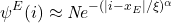where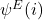is the amplitude of the wavefunction on site i,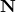is the normalizing constant, ,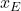is the site the wavefunction is localized around, andis the localization length.

In this simulation, we have a Hamiltonian representing particles being able to move around in space within a lattice and subject to some potential energy landscape. We write that Hamiltonian as a matrix in the computer by discretizing space—which is akin to letting the particles hop on a lattice. Next, using standard library procedures, we can diagonalize the Hamiltonian, i.e., find its eigenvalues and eigenvectors. When one writes a quantum state as a superposition of different basis vectors, the number in front of each basis vector squared is the probability of finding the particle at that point in space. By diagonalizing the Hamiltonian, we can then find the probability of the particle being at each point from the components of the eigenstates. We expect these probabilities to be decaying from a point the particle is localized around. Through this simulation, we are interested in quantifying the rate at which this decay happens. Normally, the decay is exponential in space1 . However, we are trying to determine if the decay rate is greater than an exponential rate (i.e., super exponential) as we vary the shape of the potential.

## Discretizing Space

To begin attacking this problem, we need a way of representing particles moving in space. We detail the methods we use in these next few sections. Let us consider a particle moving in space, subjected to a potential energy. We can approximate space as a discrete set of points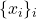where each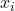is a point in a-dimensional space. For simplicity, this simulation is in one dimension, so the pointsare just real numbers. Let us further assume the points are uniformly spaced (i.e., eachis separated from its nearest neighbors by a distance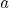, called the grid spacing). The set of points is effectively a 1d grid of the form: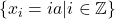where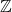is the set of integers. Typically, we work with finite grids in simulations, so thatrather than an arbitrary integer. The Hamiltonian for our quantum system may always be expressed as a matrix after a basis has been decided. There is a particularly straightforward choice of basis for a single particle traveling on a grid: the set of states in which the particle is present at a particular grid point. We denote the state in which the particle is atas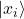. The set of’s forms a basis for the Hilbert Space of the system, which is the vector space comprised of all possible states of the quantum system. The action of the Hamiltonian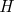on the basis states is:

(2)The numbersare the matrix elements of the Hamiltonian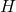in the basis; they capture how strongly the Hamiltonian “connects” the basis states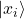and. Note that, in order to ensure the eigenvalues are real, we typically require the Hamiltonian to be symmetric; that is,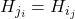. The matrix ofin the basis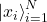is then:

(3)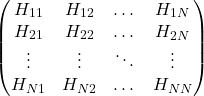We can now find the eigenvalues and eigenvectors of this matrix.

## The Hamiltonian Matrix

We now need to find the matrix elements for our system of interest. Consider a Hamiltonian of the form:

(4)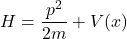where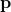is the momentum of the particle. The two terms in this Hamiltonian represent the kinetic and potential energy of the particle, respectively. In 1D quantum mechanics,is replaced by the second derivative operator:-> –On a discrete grid with spacing a, we can approximate the second derivative as:

(5)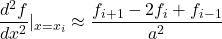where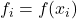is the value of \textit{f} evaluated at position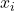. In particular, for a quantum state of the form, we have:

(6)where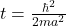.

To get the potential energy, we just multiply it by the value of the potential at each point in space. For a 1D grid, this takes the form:

(7)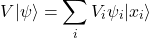where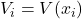. Combining the previous two equations, we get

(8)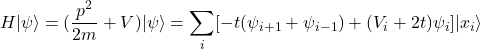Since we can always shift the potential by a constant, we can absorb theinto V, and here we can read off what the matrix elements of H will be:

(9)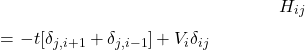where (equation 10)

(10)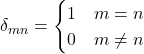is the Kronecker delta. In other words,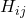is -t if i and j differ by 1 (from the kinetic energy piece), andif i and j are equal (from the potential energy piece). The matrix of the Hamiltonian is then shown in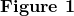.This is the Hamiltonian as a matrix assuming open boundary conditions (i.e., the values of the potential on the main diagonal, and -t on the first upper and lower diagonals.

The eigenvalues of the Hamiltonian represent the energies the system can have, and the eigenvector for each energy value represents the quantum state of the system, given as some vector in the vector space of possible states11.

## Diagonalization

Now that we have the Hamiltonian as a matrix, we can diagonalize it using any available diagonalization routine (for this simulation we will use numpy.linalg.eigh). This gives us a set of eigenvectors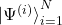with associated eigenvalues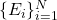, in the sense that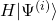=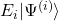.To connect this with localization, we are then interested in the components of the eigenvectors (i.e., the coefficients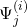in the expansion=, and how their magnitude decays in space.

Let us look at largesystems. First, we consider a system with no potential: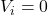for all i.shows the components of the middle eigenstate (sorted in ascending order of eigenvalue) as a function of site number i: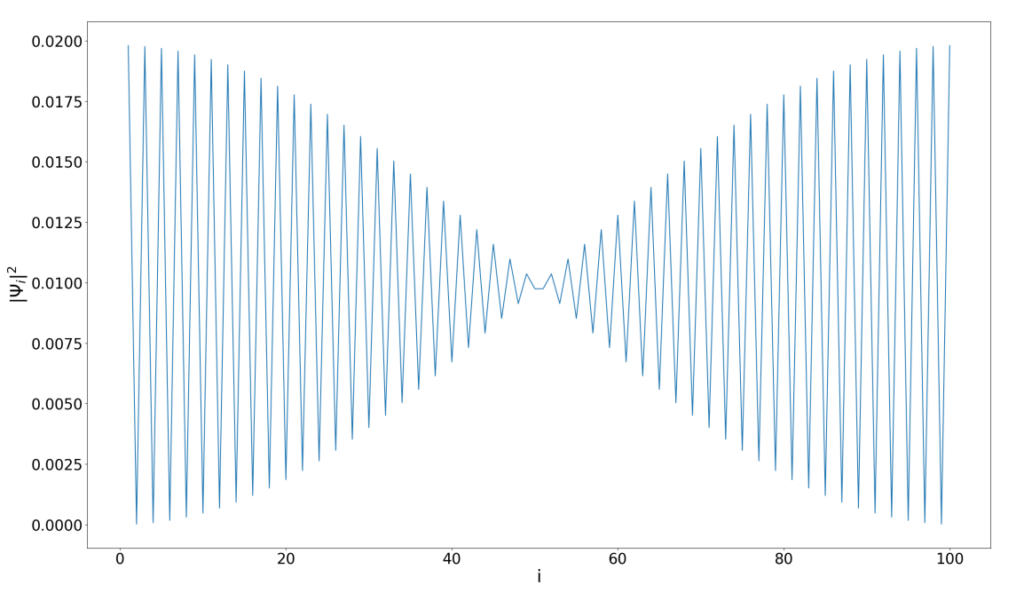A plot of the absolute square of the wavefunction amplitude versus lattice site for the middle eigenstate of a “clean” system (i.e., = for all sites). We can see that this wavefunction has an appreciable amplitude throughout the entire grid and demonstrates how non-localized particles can potentially propagate across the entire system

Let us now consider the same system, but with each Vi sampled uniformly at random between -1 and 1. An example of the center wavefunction is shown in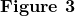Note that the wavefunction components decay quickly from the left side of the grid, and do not seep into the rest of the grid as they did with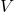=. This is precisely the phenomenon of Anderson localization—the particle remains localized on the left side of the grid when in this state.

## Localization Length

Thus far, we have been considering(i.e , eachsampled uniformly at random between -W and W). Such a potential is expected to give eigenstates that are exponentially localized:

(11)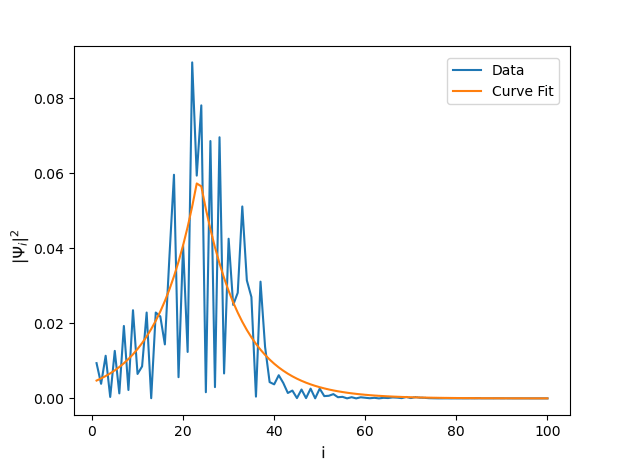A graph representing Anderson localization. Here, the wavefunction’s amplitude dies off very quickly away from the center site and has no chance of getting to the right side of the grid. The curve fit of this graph is the fit of the amplitudes to the form of equation (1)

While expected in the presence of disorder on general grounds, exponential localization may not survive the presence of interactions between particles in two or more spatial dimensions. It is then worth asking whether it is possible to localize wavefunctions superexponentially; i.e., if we can have wavefunctions of the form of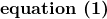with angreater then 1. This question was examined for a cosine in two dimensions and found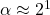. We would like to further this work and see just how “localized” we can make our wavefunctions.

To that end, Foo et al1. took the potential to be:

(12)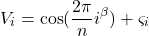where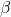is greater than 0 and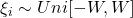, as before. This cosine term is called a confining potential. The case=was examined by Foo et al. and arises for example when one constructs so-called “optical lattices” (in which lasers are used to confine particles at certain points in space)1. Although Foo et al1. used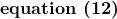for the confining potential, we used a different form of the potential to see if we get different results:

(13)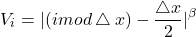An example of the confining potential used for the randomness. Here, the period is 5 and is 2. This function essentially gives a repeating pattern of powers of the distance (in x) from the various center positions

## Results

We report two major findings from the simulation: the degree of the wavefunction decay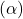decreases when the degree of the confining potential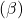increases and when the strength of the system’s disorder (W) decreases. The degreealso remains between 1 and 2. This suggests that this stabilization mechanism only works for two dimensions because we cannot push the decay higher than a Gaussian fit, as originally reported by Foo et al1.

Furthermore, we also plotted the localization length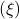as seen into verify that the system was well localized. We found the system to be quite localized for all values ofas the localization length is very small. This can be seen in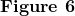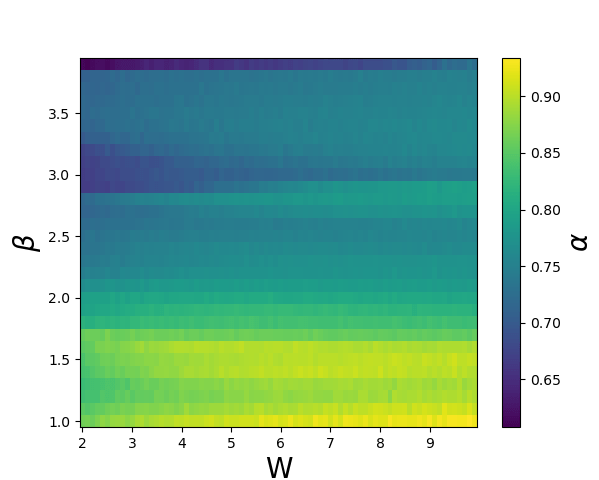A heat map that represents the degree of decay respective to the exponent of the confining potential and the disorder strength (W). was varied from 1 to 4 at intervals of 0.1 and W was varied from 2 to 10 at intervals of

## Discussion and Conclusion

The results produced prove our original hypothesis wrong. This shows that the method that we proposed does not work well, which suggests that this stabilization mechanism only works for two dimensions because we cannot push the decay higher than a Gaussian fit.This could also mean that we need to choose a better form of the confining potential in our simulation. This finding could provide valuable information and an example for future works aimed at finding such results in dimensions two and higher and with particle interactions as well. Future works could also be dedicated to investigating why this method did not work as expected.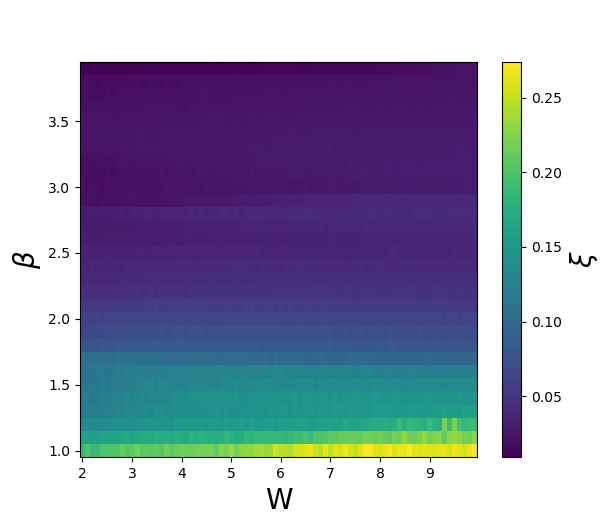A heat map that represents the localization length , which is in units of the grid spacing, versus the exponent of the confining potential and the disorder strength . was varied from 1 to 4 at intervals of and was varied from 2 to 10 at intervals of

## Acknowledgments

I would like to thank mentor Liam O’Brien from the California Institute of Technology for his guidance in the development of this research paper.